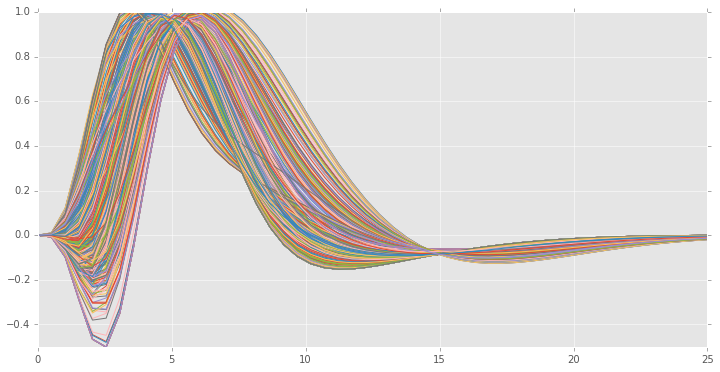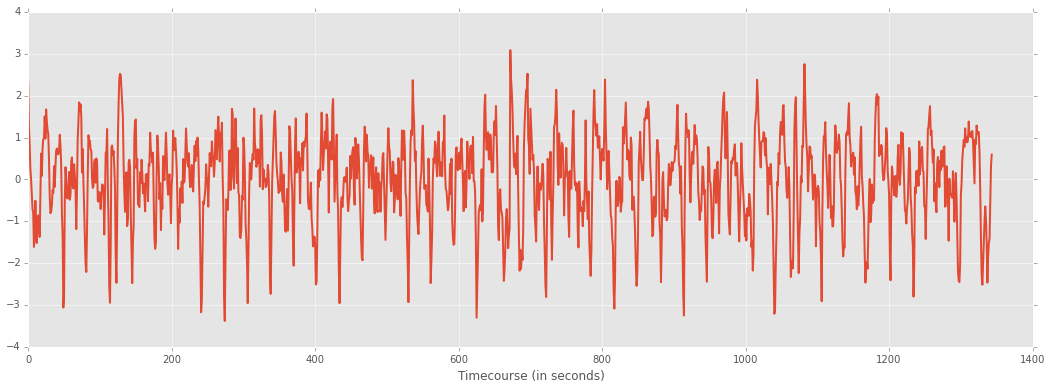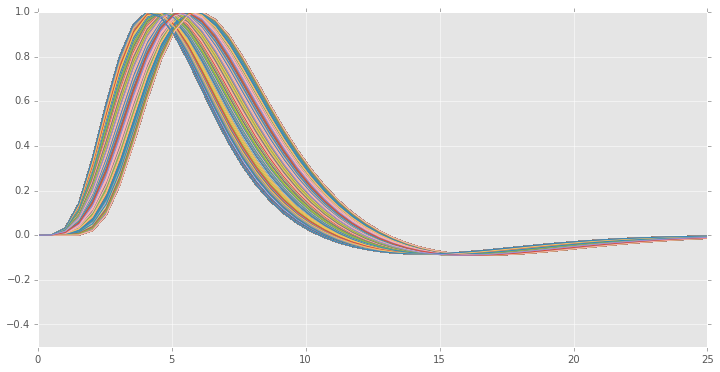# Usage example of hrf_estimation¶

Here we will use real fMRI data to estimate the HRF and activation pattern using the python package hrf_estimation.For this, we will rely on some publicly available datasets and the hrf_estimation package, which implements the estimation methods described in the publication Data-driven HRF estimation for encoding and decoding models, NeuroImage January 2015

To follow this notebook basic knowledge about fMRI signal processing is assumed.

In :
# import packages we will need
%pylab inline

# nicer plots, but only available in recent matplotlib
try:
plt.style.use('ggplot')
except:
pass

import hrf_estimation as he
print('You are running hrf_estimation version %s' % he.__version__)

Populating the interactive namespace from numpy and matplotlib
You are running hrf_estimation version 0.7


Now we will load some sample data. This data is described in the paper “Identifying natural images from human brain activity.,” K. N. Kay et al. Nature 2008, and is publicly available from http://crcns.org. See here for more details and how to obtain the full dataset: http://crcns.org/data-sets/vc/vim-1/about-vim-1 .

The following function will download the data to temporary file in the current directory. It will then load the data and perform a local detrending (Savitzky-Golay filter).

The returned data consist of an array of 500 voxel timecourses, a list of conditions representing the condition ID and a list of onseets representing the time onset of its relative condition. The list of conditions and onsets have the same length. The repetition time (TR) for this dataset is 1 second.

In :
voxels, conditions, onsets = he.data.get_sample_data(0, full_brain=False)
print('Num of timepoints in a single voxel: %s' % voxels.shape)
print('Num of voxels: %s' % voxels.shape)
print('Num of conditions: %s' % len(conditions))
print('Num of onsets: %s' % len(onsets))

Downloading BOLD signal
Num of timepoints in a single voxel: 3360
Num of voxels: 500
Num of conditions: 700
Num of onsets: 700


This data contains the BOLD time series for 1 session.

In the next step we will detrend the signal using a Savitzky-Golay filter with polynomial of degree three and window length of 121 seconds. The time series for one voxel is plotted after detrending.

In :
### perform detrending: Savitzky-Golay filter
n_voxels = voxels.shape[-1]
voxels = voxels - he.savitzky_golay.savgol_filter(voxels, 91, 3, axis=0)
voxels /= voxels.std(axis=0)
print("Performed detrending of the BOLD timecourse using a Savitzky-Golay filter")

# plot one voxel
plt.figure(figsize=(18, 6))
plt.plot(voxels[:2 * 672, 0], lw=2)
plt.xlabel('Timecourse (in seconds)')
plt.show()

Performed detrending of the BOLD timecourse using a Savitzky-Golay filter# HRF estimation with the Rank-1 GLM (R1GLM) model¶

We will proceed to estimate the HRF using a R1GLM model.

We will now call the glm function in hrf_estimation. We will use a basis formed by the HRF plus its time and dispersion derivatives. This basis is denoted '3hrf' in this package.

In :
# we construct the matrix of drifts as the matrix of ones that account for
# the intercept term. If you have drifts estimated by e.g. SPM you can add
# them here.
drifts = np.ones((voxels.shape, 1))

# we call he.glm with our data and a TR of 1 second. This will return the
# estimated HRF and the
# we also specify the basis function for the HRF estimation
hrfs, betas = he.glm(conditions, onsets, 1., voxels, drifts=drifts, mode='r1glm', basis='3hrf', verbose=1)

.. creating design matrix ..
.. done creating design matrix ..
.. computing initialization ..
.. done initialization ..
.. completed 500 out of 500 ..


The output of he.glm produces estimated HRFs and betas. For the case of 3hrf basis these have been normalized so that the resulting HRF correlates positively with the reference HRF and its maximum value is one.

We will now plot the estimated HRFs,

In :
xx = np.linspace(0, 25) # range of values for time
# construct the final HRF by multiplying by its basis
generated_hrfs = hrfs * he.hrf.spmt(xx)[:, None] + hrfs * he.hrf.dspmt(xx)[:, None] + hrfs * he.hrf.ddspmt(xx)[:, None]

plt.figure(figsize=(12, 6))
plt.plot(xx, generated_hrfs)
plt.ylim((-.5, 1.))
plt.show()Before we have used a 3hrf basis, the basis consisting of the reference HRF plus its time and dispersion derivative. If we only consider the derivative with respect to time we obtain the 2hrf basis. We now fit the same model using a 2hrf basis instead and plot the estimated HRFs

In :
hrfs, betas = he.glm(conditions, onsets, 1., voxels, drifts=drifts, basis='2hrf', verbose=1)
xx = np.linspace(0, 25) # range of values for time
# construct the final HRF by multiplying by its basis
generated_hrfs = hrfs * he.hrf.spmt(xx)[:, None] + hrfs * he.hrf.dspmt(xx)[:, None]

plt.figure(figsize=(12, 6))
plt.plot(xx, generated_hrfs)
plt.ylim((-.5, 1.))
plt.show()

.. creating design matrix ..
.. done creating design matrix ..
.. computing initialization ..
.. done initialization ..
.. completed 500 out of 500 ..In :
hrfs, betas = he.glm(conditions, onsets, 1., voxels, drifts=drifts, basis='fir', verbose=1)
xx = np.linspace(0, 25) # range of values for time
# construct the final HRF by multiplying by its basis
# generated_hrfs = hrfs * he.hrf.spmt(xx)[:, None] + hrfs * he.hrf.dspmt(xx)[:, None]

plt.figure(figsize=(12, 6))
plt.plot(np.arange(hrfs.shape), hrfs)
plt.ylim((-.5, 1.))
plt.axis('tight')
plt.show()

.. creating design matrix ..
.. done creating design matrix ..
.. computing initialization ..
.. done initialization ..
.. completed 500 out of 500 ..